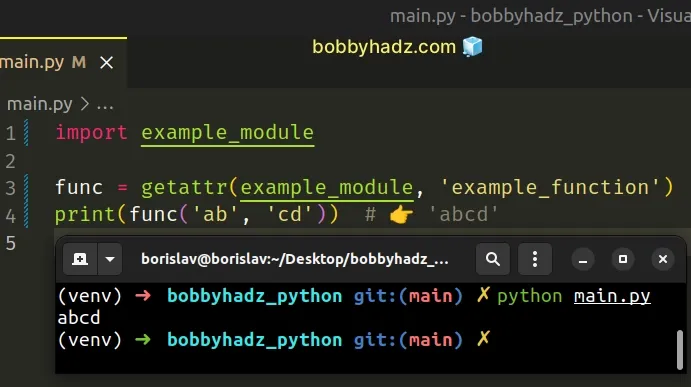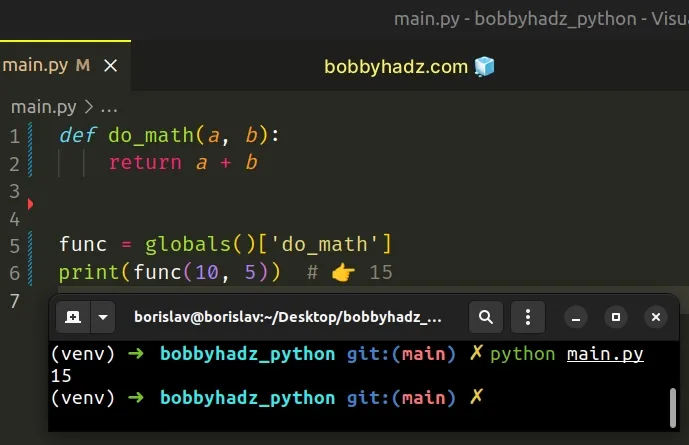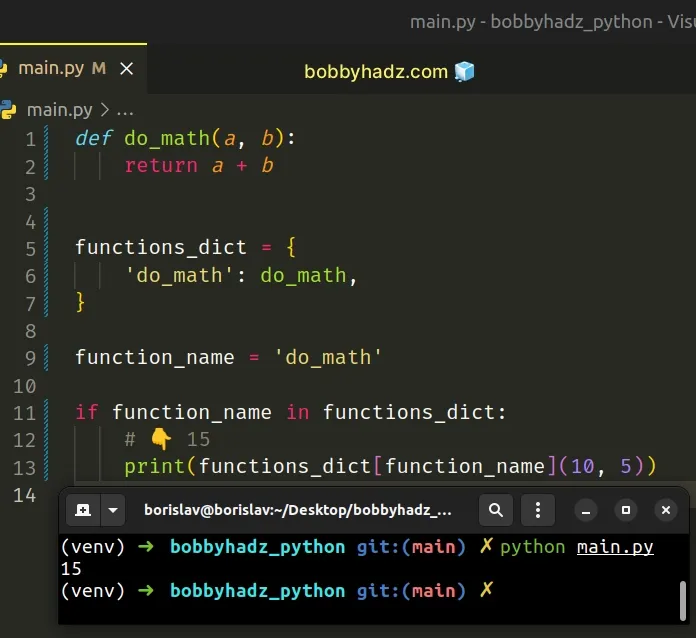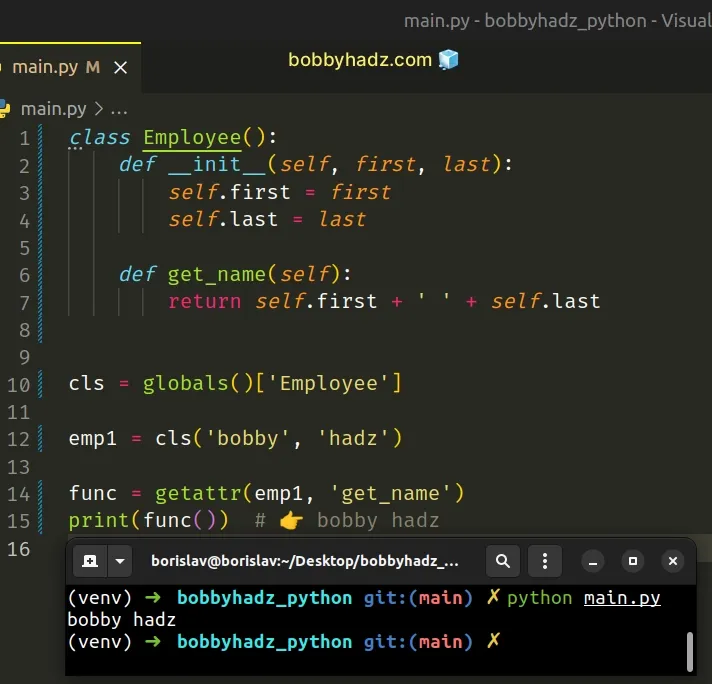# Call a Function by a String name in PythonLast updated: Feb 22, 2023
6 min## #Call a function by a String name in Python

To call a function by a string name:

1. Import the module the function is defined in.
2. Use the `getattr()` function to get the function.
3. Call the function.

If you need to call a function given a string name and the function is defined in a different module, you first have to import the module.

main.py
```Copied!```import example_module

func = getattr(example_module, 'example_function')
print(func('ab', 'cd'))  # 👉️ 'abcd'
``````

The code sample assumes that there is a module named `example_module` located in the same directory.

example_module.py
```Copied!```def example_function(a, b):
return a + b
``````Once we have the module that defines the function imported, we can use the `getattr()` function to get the function by a string name.

The getattr function returns the value of the provided attribute of the object.

The function takes the following parameters:

NameDescription
objectthe object whose attribute should be retrieved
namethe name of the attribute
defaulta default value for when the attribute doesn't exist on the object

The `getattr()` function will return the function, so the last step is to invoke it and provide all the required arguments.

main.py
```Copied!```import example_module

func = getattr(example_module, 'example_function')
print(func('ab', 'cd'))  # 👉️ 'abcd'
``````

If the function is defined in the same module, use the `globals()` function.

## #Call a function by a String name using globals()

This is a three-step process:

1. Use the `globals()` function to get a dictionary containing the current scope's global variables.
2. Use the string as a dictionary key to get the function.
3. Call the function.
main.py
```Copied!```def do_math(a, b):
return a + b

func = globals()['do_math']
print(func(10, 5))  # 👉️ 15
``````The globals function returns a dictionary that implements the current module namespace.

main.py
```Copied!```def do_math(a, b):
return a + b

# {'__name__': '__main__', '__doc__': None, '__package__': None, '__loader__': <_frozen_importlib_external.SourceFileLoader object at 0x7f151f799de0>, '__spec__': None, '__annotations__': {}, '__builtins__': <module 'builtins' (built-in)>, '__file__': '/home/borislav/Desktop/bobbyhadz_python/main.py', '__cached__': None, 'do_math': <function do_math at 0x7f151f8e3d90>}
print(globals())
``````

You can use the string to access the specific key in the dictionary.

There is a key with the function's name that points to the actual function.

If a function with the given name doesn't exist in the module, you'd get a `KeyError` exception.

The last step is to use parentheses to call the function providing it any arguments it requires.

## #Call a function by a String name using a dictionary

Alternatively, you could define a dictionary that maps function names to the actual functions.

main.py
```Copied!```def do_math(a, b):
return a + b

functions_dict = {
'do_math': do_math,
}

function_name = 'do_math'

if function_name in functions_dict:
# 👇️ 15
print(functions_dict[function_name](10, 5))
``````The `functions_dict` dictionary has function names as keys and the actual functions as values.

If `if` statement checks if the specified string is present in the dictionary before calling the function.

You can use the `getattr()` function if you need to call a class method by a string name.

## #Call a class method by a String name in Python

To call a class method by a string name:

1. Instantiate the class to get an object.
2. Use the `getattr()` function to get access to the method.
3. Call the method.
main.py
```Copied!```class Employee():
def __init__(self, first, last):
self.first = first
self.last = last

def get_name(self):
return self.first + ' ' + self.last

cls = globals()['Employee']

func = getattr(emp1, 'get_name')
``````If you have to call a method by a string name from within another class method, you would pass `self` as the first argument to the `getattr()` function.

If the class is defined in a different module, you would have to import it.

You can either import the class directly or use the `importlib.import_module()` method.

## #Importing a class and calling a method by string name

Here is an example that imports the class directly and calls a method by a string name.

main.py
```Copied!```import example_module

cls = getattr(example_module, 'Employee')

func = getattr(emp1, 'get_name')
``````

The code sample assumes that there is a module named `example_module` located in the same directory.

example_module.py
```Copied!```class Employee():
def __init__(self, first, last):
self.first = first
self.last = last

def get_name(self):
return self.first + ' ' + self.last
``````

We used the `getattr()` function to get the class and then used the function to get the method.

The last step is to invoke the method.

## #Using `importlib` to import the module before calling the method by string name

Here is an example that uses the `importlib.import_module()` method to import the module before calling a specific method given a string name.

main.py
```Copied!```import importlib

module = importlib.import_module('example_module')

cls = getattr(module, 'Employee')

func = getattr(emp1, 'get_name')
``````

The code sample assumes that there is a module named `example_module` in the same directory and the module defines an `Employee` class.

The importlib.import_module method takes the name of a module and imports it.

The `name` argument can be absolute or relative, e.g. `pkg.module` or `..module`.

If you use a relative package name, e.g. `..module`, you have to pass a second argument to the `import_module()` method, e.g. `import_module('..module', pkg.subpkg')` imports `pkg.module`.

## #Call a function by a String name using locals()

If the function you need to call is located in the same module, you can also use the `locals()` dictionary.

main.py
```Copied!```def example_function(a, b):
return a + b

# 👇️ 105
print(
locals()['example_function'](50, 55)
)
``````

This approach is similar to using the `globals()` dictionary, however, the `locals()` function returns a dictionary that contains the current scope's local variables, whereas the `globals` dictionary contains the current module's namespace.

## #Using globals() vs locals() to call a function by string name

Here is an example that demonstrates the difference between the `globals()` and the `locals()` dictionaries.

main.py
```Copied!```def example_function():
return 'GLOBAL example function'

def call_function_by_string_name():
def example_function():
return 'LOCAL example function'

# 👇️ GLOBAL example function
print(globals()['example_function']())

print('-' * 30)

# 👇️ LOCAL example function
print(locals()['example_function']())

call_function_by_string_name()
``````

The function with the name `example_function` is defined in the global and local scopes.

When we used the `globals()` dictionary, we called the global function (the one from the outer scope).

When we used the `locals()` dictionary, we called the local function (the one that is defined inside the function).

## #Call a function by a String name using eval()

You can also use the `eval()` function to call a function by string name.

main.py
```Copied!```def greet(name):
return f'hello {name}'

function_name = 'greet'

func = eval(function_name)
print(func)  # 👉️ <function greet at 0x7f7e978504a0>

``````

The eval function takes an expression, parses it and evaluates it as a Python expression using the `globals` and `locals` dictionaries as the global and local namespace.

The `eval()` function should only be used with trusted code. Don't use `eval()` with user-generated data.

The `eval()` function basically tries to run the given expression, so it should never be used with untrusted code.

## #Call a function by a String name using exec()

You can also use the `exec()` function to call a function by string name.

main.py
```Copied!```def greet(name):
return f'hello {name}'

function_name = 'greet'

exec(f'my_func={function_name}')

print(my_func)  # 👉️ <function greet at 0x7f7e978504a0>

``````
Make sure to only use this approach if you can trust the data stored in the dictionary. Never use the `exec()` function with untrusted user input.

The exec function supports dynamic execution of Python code.

The function takes a string, parses it as a suite of Python statements and runs the code.

We assigned the result of evaluating the string name to the `my_func` variable.

You can then use the `my_func` variable to invoke the function as shown in the code sample.

When using this approach, you are likely to get linting warnings because the variable is declared implicitly using `exec()`.